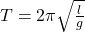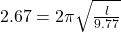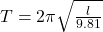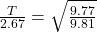## A pendulum that has a period of 2.67000 s and that is located where the acceleration due to gravity is 9.77 m/s2 is moved to a location wher

Question

A pendulum that has a period of 2.67000 s and that is located where the acceleration due to gravity is 9.77 m/s2 is moved to a location where it the acceleration due to gravity is 9.81 m/s2. What is its new period? (Enter your answer in seconds and to at least 5 decimal places.)

in progress 0
4 weeks 2021-08-17T12:55:41+00:00 1 Answers 3 views 0

Explanation:

Expression for time period of pendulum is given as followswhere l is length of pendulum and g is acceleration due to gravity .

Putting the given values for first placePutting the values for second placeDividing these two equationT = 2.66455 s.